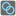• # What am I missing?

## Question related to missionBreak Rings

My code is here, but I cannot pass this assertion.

```break_rings(((1,3),(3,4),(3,5),(4,6),(6,7),(8,3),(8,1),(2,6),(8,4),(9,5),(4,5),(1,7),))
```

My answer is 5, but my result is 6. I guess I miss some conditions.

```def find_the_nuisance(box):
max_len=0
max_len_num=0
for k, v in box.iteritems():
if max(max_len, len(v)) == len(v):
max_len=len(v)
max_len_num=k
if max_len == 0:
return 0
else:
return max_len_num

def break_the_chain(nuisance, box):
if nuisance == 0:
return False
else:
friend=[]
friend=box.pop(nuisance)
#print friend
for n in friend:
box[n].remove(nuisance)
print box
return True

def break_rings(rings):
import collections
box=collections.defaultdict(list)
for ring in rings:
x, y = ring
box[x].append(y)
box[y].append(x)
print box
boool=True
count=0
while boool:
nuisance = find_the_nuisance(box)
print nuisance
boool = break_the_chain(nuisance, box)
count+=1
return count-1
```